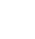# Excel Match Formula

The ‘Match’ formula could be described as a versatile relative of the Vlookup and Hlookup functions. Whilst vlookup can only find corresponding data to the right of the lookup data, match can help you to return information from any direction using it’s friendly assistant, ‘Offset’.

Match also has it’s own unique abilities and there are lots of instances where you would use ‘Match’ instead of trying to find data using vlookup. Match will search for your value (or a greater than / less than) in a list vertically or horizontally and return the position of the searched value – the Match.

This is especially useful in many situations, some very basic and some very complex. We’ll deal with the more basic parts in this blog but I will mention a few other more complex uses just to give you a bit of an insight or inspiration for your Excel project or predicament.

Like all formulas, it starts with = and then the formula name and the brackets that then contain the logic.

Similar to vlookup it starts with the value you want to find followed by the range or ‘area of interest’ then the type of match. This example will deal with the end logic of 0 (zero) which means an exact match. The other options are 1 or -1 which are used primarily in matching nearest numbers whether over or under if it can’t match exactly.

The image below shows how this works. So if we want to find out some stuff about Apples in our data which could be hidden amongst thousands of items in a list we would use match to identify where on the list Apples were from the top row, in this example B1 is the top row.

``` =Match(D11,B1:B7,0) ```This would give us a result of 3. Including the top row where we started, Apples are 3 rows down. Now we know where the data for apples is on our dataset we can use that number to obtain other information from that row that relates directly to apples by using ‘Match’ friend ‘Offset’ or by using other formulas or even going and physically looking in row 3 if that’s what we need to do.

This only seems like ‘half a job’ done as first thoughts are so what !

The power becomes apparent when you use the result of the match in other formulas and just as an example (each will be covered in other blogs):

Offset – This function will allow you to return the information anywhere surrounding the match – left, right, up, down and by as many rows or columns as you instruct.

Match – Using the location of the matched value as the starting point in your next match for example to find the 2nd instance of the same value.

Indirect – Use the location of the match in the indirect formula.

Countif – Use the matched value location to determine a range that you need to count specific values in.

We deal with these examples and other more complex ‘Match’ formulas with examples in some of our other blogs. You can use the site search facility to find them.

Tips for Match

Errors may occur where you are looking to match a number that is stored as text on your worksheet. Because the 2 will appear different to Excel we need to use the TEXT formula but feel free to ask us a question if you need help on any of this or leave a comment for us to answer so everyone can see the answer.